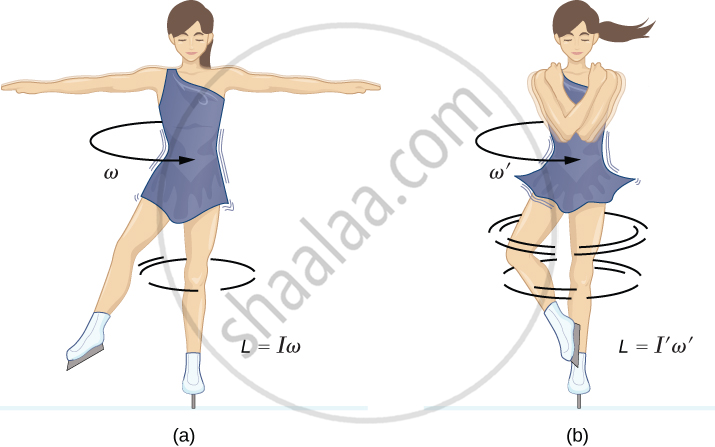Department of Pre-University Education, KarnatakaPUC Karnataka Science Class 11

# Angular Momentum in Case of Rotation About a Fixed Axis

## Notes

### Angular Momentum In Case of Rotation about a Fixed Axis:

We know that "L" = "r"xx"p"

"L"="rx"("mv")

Now, "v"=omega"r"        ⇒"L"=mr^2omega

"L" = "I"omega

Connservation of angular momentum:

We know that, "dL"/"dt"=d/dt("I"omega)="r"

If tau_"ext"=0  "then"  "I"omega="constant"Example:

• When a person is rotating with hands stretched, so the moment of inertia will be more because distribution of mass is far from the axis of rotation.

• As the person brings his arms close to body, moment of inertia decreases because the mass is now distributed close to axis.

• In this situation no external torque is applied, means angular momentum is conserved.

• Iω = constant.

• As I decrease, angular velocity ω

Example- A child stands at the centre of a turntable with his two arms outstretched. The turntable is set rotating with an angular speed of 40 rev/min. How much is the angular speed of the child if he folds his hands back and thereby reduces his moment of inertia to 2/5times the initial value? Assume that the turntable rotates without friction.

Solution: When the child folds his hand back, there is no external torque involved, so the angular momentum is conserved in this case.

We can apply the equation

Iomega="constant"

Iomega=(2/5I)omega'

Hence we can find omega

If you would like to contribute notes or other learning material, please submit them using the button below.

### Shaalaa.com

Angular momentum of Rotating Body [01:19:55]
S
0%